# 1720 Transforming Puzzle-A-Day’s Wizard’s Hat

### Today’s Puzzle:

I recently saw a fun-to-solve puzzle from Puzzle a Day on Twitter:

The puzzle got me thinking, what would happen if the numbers weren’t from 1 to 10, but were from 0 to 9 instead? Would it change the puzzle a little or a lot?It actually changes the puzzle quite a bit. There are a lot more solutions than for the Puzzle a Day puzzle! And I don’t mean just switching the red line with the green line and/or switching the purple circles at the bottom. How many solutions can you find?

### Factors of 1720:

• 1720 is a composite number.
• Prime factorization: 1720 = 2 × 2 × 2 × 5 × 43, which can be written 1720 = 2³ × 5 × 43.
• 1720 has at least one exponent greater than 1 in its prime factorization so √1720 can be simplified. Taking the factor pair from the factor pair table below with the largest square number factor, we get √1720 = (√4)(√430) = 2√430.
• The exponents in the prime factorization are 3,1 and 1. Adding one to each exponent and multiplying we get (3 + 1)(1 + 1) (1 + 1) = 4 × 2 × 2 = 16. Therefore 1720 has exactly 16 factors.
• The factors of 1720 are outlined with their factor pair partners in the graphic below.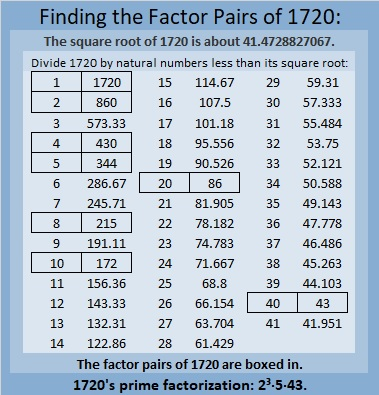### More About the Number 1720:

1720 is the difference of two squares in FOUR different ways:
431² – 429² = 1720,
217² – 213² = 1720,
91² – 81² = 1720, and
53² – 33² = 1720.

# 1719 A Meaningful Statistics Project

### What Is This Statistics Project?

FamilySearch.org and Ancestry.com have a statistics project that you can be involved with. It’s indexing the 1950 census. This project is unlike any other project I’ve seen. A computer has already indexed the census, and they just want humans to verify that the computer did it right. Also not only can you choose the state, but also the surnames that you verify. The project has been going on for about three months already, but I didn’t look into it until the middle of May.  My home state, Nevada, is 100% done so I missed out on verifying my family’s data. Instead, I tried to find my husband’s family. They lived in Ohio, but some of them moved to California in 1950. I wasn’t sure which month they moved. The program asked me if I wanted to find a particular surname. I chose Sallay a few times in both Ohio and California, and I indexed whatever Sallay person I saw and their entire household. Sometimes I did their neighbors, but most of the time I didn’t. On about the tenth try, this page came up: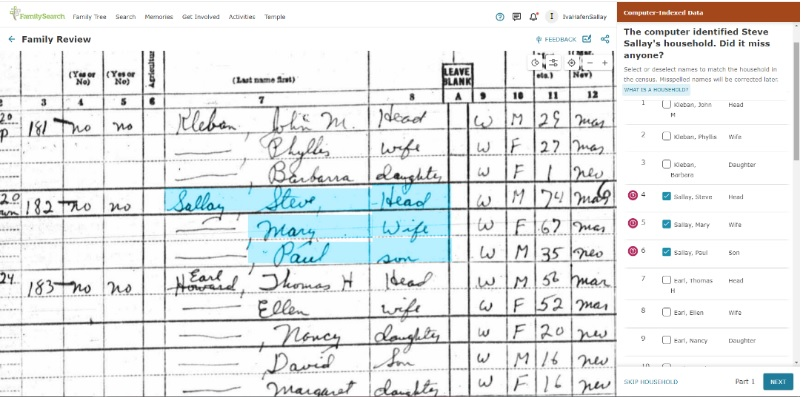I was so thrilled. The family in blue is my husband’s grandfather, grandmother, and Uncle Paul.  His grandparents had died before I ever met my husband, but I have read his grandfather’s journal, and I feel like I know him and his wife pretty well. My husband’s Uncle Paul was very near and dear to my heart. For twenty years he was always very kind to my family whenever we visited him, and it was my privilege to move him into my home and be his primary caregiver for the last 7 1/2 years of his life.

I did not index the record. I had my husband do it. It was a sweet experience. Perhaps YOU have family members who were alive in the United States in 1950 that you could index. It could be one of the most meaningful statistics projects of your life!

How did the computer do indexing my husband’s family members? It got their names right, but it completely got their street name wrong. Since I knew the street name, I was able to change it from something like Ainberland to the correct name of Cumberland.

My father, mother, two sisters, and a brother are in the 1950 Census. They lived in the house that I would come home to shortly after my birth in a few more years. The thing that amazed me the most was our house number. I’ve known the street name my entire life, but I didn’t know the house number until today. For the first 5 years of my life, my house number was 2535, and that also just happens to be my house number for these last 28 1/2 years, too, and I don’t have plans to move anytime soon. I was so stunned at this revelation, that I called my sister who has been to my house many times. She knew the house numbers were the same, but never mentioned it to me because she figured I was so good with numbers that I already knew.

What will surprise you when you look at the 1950 Census? Indexing your own family can prevent errors. I wish I had indexed my family. I would not have said my sister was already a widow at five years of age or that my brother was born in Cavada. Find your family in the census and get their information right!

Now I’ll write a little about the number 1719 because this is my 1719th post.

### Factors of 1719:

• 1719 is a composite number.
• Prime factorization: 1719 = 3 × 3 × 191, which can be written 1719 = 3² × 191.
• 1719 has at least one exponent greater than 1 in its prime factorization so √1719 can be simplified. Taking the factor pair from the factor pair table below with the largest square number factor, we get √1719 = (√9)(√191) = 3√191.
• The exponents in the prime factorization are 2 and 1. Adding one to each exponent and multiplying we get (2 + 1)(1 + 1) = 3 × 2 = 6. Therefore 1719 has exactly 6 factors.
• The factors of 1719 are outlined with their factor pair partners in the graphic below.### More About the Number 1719:

1719 is the difference of two squares in three different ways:
860² – 859² = 1719,
288² – 285² = 1719, and
100² – 91² = 1791.

1791 is the sum of nine consecutive odd numbers:
183 + 185 + 187 + 189 + 191 + 193 + 195 + 197 + 199 = 1719.
The numbers in red are prime numbers that form a prime decade.

# The 153rd Playful Math Education Blog Carnival!

Welcome to the 153rd Playful Math Education Carnival! Thanks to those who blogged and/or tweeted about math, we have another fun-filled carnival this month. Since a picture is worth 1000 words, and tweets usually have lovely pictures and captions included with them, I’ve embedded a lot of tweets in this carnival. Many of the tweets include links to blog posts. You can be transported directly to any area of the carnival you desire by clicking one of the following links:

### Ukraine and Math

I have been very upset about the recent events in Ukraine and wondered how I could possibly publish a cheery, playful carnival at this time.

I decided to publish the carnival but include a couple of blog posts that link math and Ukraine.

This first post is a poem about the current situation: Evil Adds Up.

The second post is about Voroinoi Diagrams and Ukrainian mathematician, Georgii Voronoi. I am glad to know a little bit about Goergii Voronoi now after reading that post.

### A Fishing Pond

You can learn some fun math facts by reading blogs. A few years ago I read a post on the Math Online Tom Circle blog that made the number 153 unforgettable for me. 153 is known as the St. Peter Fish Number.

Now fishing pond booths are often a part of a traditional carnival so this 153rd edition of the Playful Math Education Blog Carnival just has to have one, too. Its fishing booth has 153 fish in it representing the 153 fish Simon Peter caught in John 21: 9-14.I made the fish tessellate because tessellation is a cool mathematical concept. The fish form a triangle because 153 is a triangular number. I colored the fish to show that
153 = 5! + 4! + 3! + 2! + 1! I like that 5! is also a triangular number so I put it at the top of the triangle, but 1! and 3! are triangular numbers, too. Can you use addition on the graphic to show that 153 = 1³+5³+3³?

### A Little Magic:

That same Tom Circle blog post also revealed the magician’s secret behind a potential math magic trick:
Pick a number, ANY number. Multiply it by 3. Then find the sum of the cubes of its digits. Find the sum of the cubes of the digits of that new number. I might have you repeat that last step a few times. I predict your final number is. . .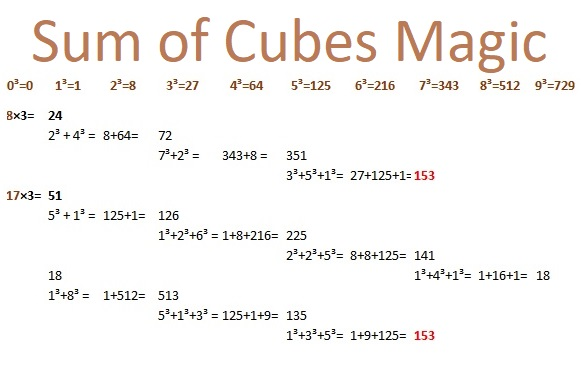No matter what number you choose, I can accurately predict what your final number will be. If you open the sealed envelope in my hand, you will see that I did indeed predict your final number, 153.

Pat’s Blog teaches about another magical number property in Squares That Parrot Their Roots.

Creative Learning AfrikA+ writes about the secret of performing well on tests in It’s not Math Magic, It’s Consistency.

### The Enchanted Tweeting Room

Speaking of the number 153, Jo Morgan recently published her 153rd Mathsgem post with many ideas from the Twitterverse:

In her 95th Monday Must-Reads blog post, Sara Carter shared some great ideas she saw on Twitter: a math word wall, some Desmos Gingerbread Houses, a Find-the-Imposter Spiderman Surds activity, A Polynomial Two Truths and a Lie game, and much more.

### Math Art

Leonardo DeVinci and many other famous artists were also famous for their mathematics. Mathematics used to be considered a liberal art. Denise Gaskins encourages us to bring back the joy of learning math in part one of Rediscover the Liberal Art of Mathematics.

RobertLovesPi uses enneagrams, regular hexagons, and other polygons to make a lovely artistic design. He also creates a shimmering 3-D shape in A Faceted Rhombicosidocecahedron with 540 Faces.

Paula Beardell Krieg wrote about the experience of directly teaching paper folding and indirectly teaching mathematics over zoom for six weeks in A Lovely Experiment.

We can use Desmos to create stunning artwork:

### Math Games

Every week Denise Gaskins shares a new game on her blog post Math Game Monday.

Julie Naturally shares some Awesome Free Math Games for Kindergartners, no electronics required.

Children at St Margaret’s Lee Church of England Primary School have been playing a domino game called the Mexican Train game. They like it so much that they’ve expressed the desire to play it at home with their families.

How much do children enjoy playing mathy board games? Just read this post by Jenorr73 of One Good Thing: Math Game Joy.

Ben Orlin of Math with Bad Drawings wrote about one of my favorite games, SET, in A Theory of Trios.

Claire Kreuz of NBC’s KARK.com blog reports that a 14-year-old High School student has developed a math game everyone can play even those with special needs.

### Puzzles

Colleen Young blogs about a new publication by Jonny Griffiths in A-Level Starters.

This puzzle is my contribution to the carnival: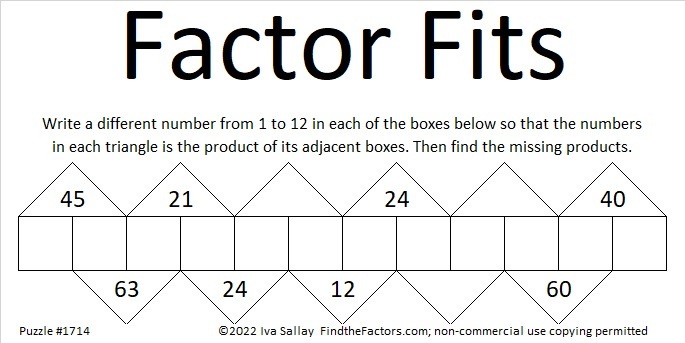Here are some puzzles I found on Twitter:

Sarah Carter regularly shares puzzles that help us play with math:

### Children’s Literature

You will want to read the responses to this next tweet. MANY biographies of mathematicians are mentioned:

### Mathematical Poetry

What’s a Fib? It’s a poetry style beautifully explained by the Kitty Cats blog.

Molly Hogan of Nix the Comfort Zone wrote thoughtful poems about the Number Zero and How Many Snowflakes Were Seen out her window.

Catherine Flynn of Reading to the Core taught me about the Fibonacci style of writing poems in Poetry Fridays: Fractals, Fibonacci, and Beyond.

Count the syllables in Heidi Mordhorst of My Juicy Little Universe’s poem Jealousy and you will have counted down from nine to one.

MaryLee Hahn of A(another) Year of Reading makes us ponder our footprint in The Mathematics of Consumerism.

### Number Sense

The Year One Class had a wonderful time playing with numbers as they put the numbers from 0 to 50 in their proper places on a number line and talked about number patterns.

Norah Colvin (Live, Laugh, Learn . . . Create the Possibilities blog) used easily stackable pancakes to help students have a better sense of how much 1000 is.

In Important ideas about addition, Tad Watanabe reminds us that children don’t necessarily understand concepts such as 30 being three tens. Students sometimes erroneously think of multi-digit numbers as “simply a collection of single-digit numbers that are somehow glued together.” He talks about what to do about these and a few other issues students face learning mathematics.

Jenna Laib of Embrace the Challenge observed that one of her students was having difficulty understanding negative numbers. Read what happened when she played a Tiny Number Game with her.

### Geometry

Look at the pictures. You can tell that Mrs. Bracken’s class enjoyed exploring and discussing ways to display four squares and their reflections.

Check out Pat’s Blog So You Thought You Knew Everything About Equilateral Triangles.

### Health and First Aid Station

Math Anxiety can be a real health issue:

Math Teachers can experience a different type of Math Anxiety:

### The Math Teacher Experience

What if a math lesson is fun but the concepts won’t be a major part of the end-of-year test? Pay attention to Melissa D of the Dean of Math blog post, It’s a fun unit, but it’s not really necessary.

Anna of iamamathteacher.blogspot.com shares how her school year has gone so far.

### Football and Math

In Inequalities on the Gridiron, Dick Lipton and Ken Regan talk about why the Buffalo Bills weren’t in the Superbowl and whether or not the c in the inequality
a² – b² = c is positive or negative.

Check out Eric Eager’s article: Football’s lessons about mathematics, academia, and industry.

The Quillette has an interesting, although possibly controversial read: It’s Time to Start Treating High School Math Like Football.

If a math student, football player, or anyone else feels like a zero, they could benefit from Fran Carona inspiring You Are Not a Zero about Cooper Kupp, the Super Bowl MVP.

### Statistics

Stanleyavestaff room 6 students enjoyed the lollipop statistics assignment so much fun that they didn’t even know they were doing math.

Joseph Nebus of Another Blog, Meanwhile made a humorous pie chart in Statistics Saturday: My Schedule for Doing Things. Many students and even adults can probably relate to it.

### Calculus and Higher Math

Joseph Nebus of Nebusresearch has been reading a biography of Pierre-Simon LaPlace, so naturally, he blogs about monkeys, typewriters, and William Shakespeare in Some Progress on the Infinitude of Monkeys.

### Math Wordle

Wordle has recently taken the world by storm. Got some math vocabulary words for your students? No matter how long the words are, your students can try to guess such words when they’re presented as wordles that you’ve made with the help of mywordle.strivemath.com. I made the one below. I told my son it was a math term and asked him to solve it:There are also numerous wordles based on numbers rather than letters:

### Stand-up Comedy Show

Archon’s Den shared some clever Math One-liners that will make you and your students either roll on the floor with laughter or roll your eyes.

### Other Carnivals

The 201st edition of the Carnival of Mathematics can be found at the team at Ganit Charcha.

Last month the 152nd Playful Math Carnival was hosted by Denise Gaskins. Perhaps you would like to host the next carnival or one later in the year. We need more volunteers! To volunteer to host the carnival go to Denise Gaskins’ Carnival Volunteer Page.

# 1718 Factor Fits Valentine

### Today’s Puzzle:

Happy Valentine’s Day! I hope you enjoy my Valentine to YOU!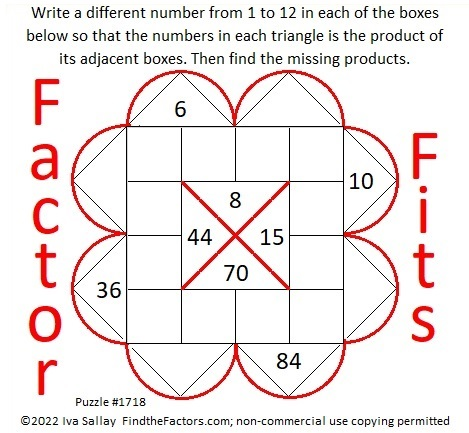### Other Mathematical Valentines:

Colleen Young of Mathematics, Learning and Technology has a nice collection of mathematical hearts For Valentine’s Day.

### Factors of 1718:

• 1718 is a composite number.
• Prime factorization: 1718 = 2 × 859.
• 1718 has no exponents greater than 1 in its prime factorization, so √1718 cannot be simplified.
• The exponents in the prime factorization are 1 and 1. Adding one to each exponent and multiplying we get (1 + 1)(1 + 1) = 2 × 2 = 4. Therefore 1718 has exactly 4 factors.
• The factors of 1718 are outlined with their factor pair partners in the graphic below.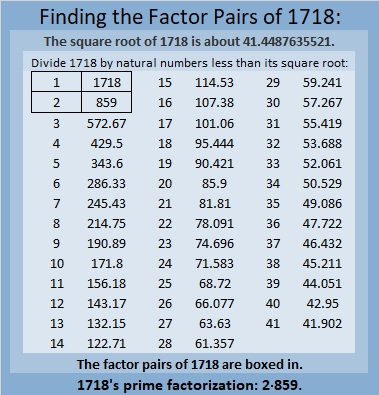### More About the Number 1718:

1718 is the sum of four consecutive numbers:
428 + 429 + 430 + 431 = 1718.

# 1717 Factor Fits for Your Valentine

### Today’s Puzzle:

Solve this Valentine-themed Factor Fits puzzle with both logic and heart!### Factors of 1717:

• 1717 is a composite number.
• Prime factorization: 1717 = 17 × 101.
• 1717 has no exponents greater than 1 in its prime factorization, so √1717 cannot be simplified.
• The exponents in the prime factorization are 1 and 1. Adding one to each exponent and multiplying we get (1 + 1)(1 + 1) = 2 × 2 = 4. Therefore 1717 has exactly 4 factors.
• The factors of 1717 are outlined with their factor pair partners in the graphic below.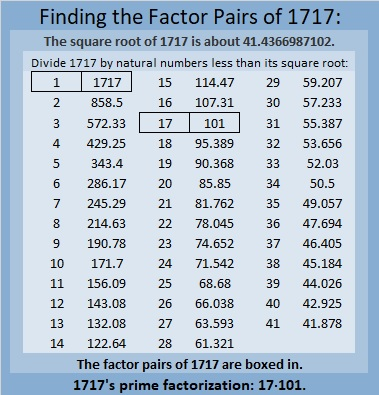### More About the Number 1717:

1717 is the sum of two squares two different ways:
41² + 6² = 1717, and
39² + 14² = 1717.

1717 is the hypotenuse of FOUR Pythagorean triples:
340 1683 1717, which is 17 times (20-99-101),
492 1645 1717, calculated from 2(41)(6), 41² – 6², 41² + 6²,
808 1515 1717, which is (8-15-17) times 101, and
1092 1325 1717, calculated from 2(39)(14), 39² – 14², 39² + 14².

# Two Factor Pairs of 1716 Make Sum-Difference!

### Today’s Puzzle:

How many factor pairs does 1716 have? Twelve. The factors in one of those factor pairs add up to 145 and the factors in a different one subtract to 145. If you can find those two factor pairs, then you can solve this puzzle!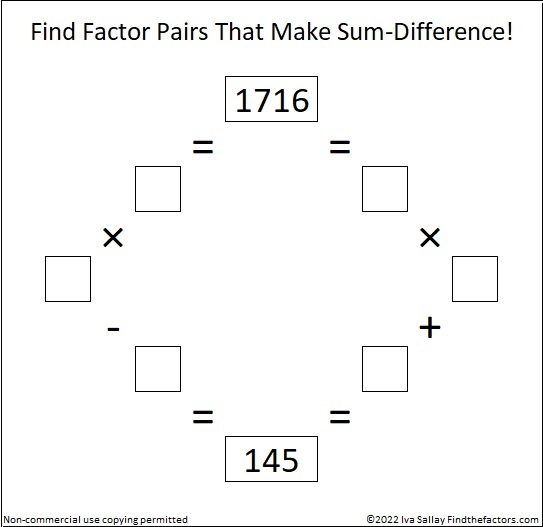Try to solve it all by yourself, but if you need some help, you can scroll down to learn more about the factors of 1716.

### A Factor Tree for 1716:

3 is a factor of 1716 because 1 + 7 + 1 + 6 = 15, a multiple of 3.
11 is a factor of 1716 because 1 – 7 + 1 – 6 = -11.

3 ·11 is 33. Here is one of MANY possible factor trees for 1716. This one uses the fact that 33 · 52 = 1716: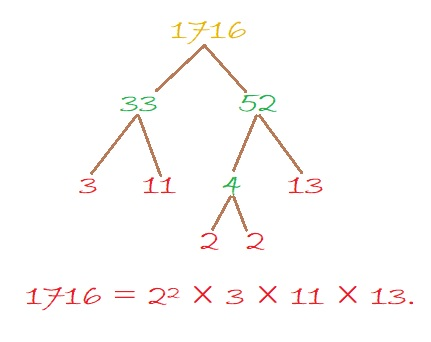### Factors of 1716:

• 1716 is a composite number.
• Prime factorization: 1716 = 2 × 2 × 3 × 11 × 13, which can be written 1716 = 2² × 3 × 11 × 13.
• 1716 has at least one exponent greater than 1 in its prime factorization so √1716 can be simplified. Taking the factor pair from the factor pair table below with the largest square number factor, we get √1716 = (√4)(√429) = 2√429.
• The exponents in the prime factorization are 2, 1, 1, and 1. Adding one to each exponent and multiplying we get (2 + 1)(1 + 1)(1 + 1)(1 + 1) = 3 × 2 × 2 × 2 = 24. Therefore 1716 has exactly 24 factors.
• The factors of 1716 are outlined with their factor pair partners in the graphic below.Here’s a chart of those same factor pairs but in reverse order with their sums and differences included.### More About the Number 1716:

1716 is the hypotenuse of a Pythagorean triple:
660-1584-1716, which is (5-12-13) times 132.

OEIS.org reminds us that 1716 is in the 6th and 7th columns of the 13th row of Pascal’s Triangle: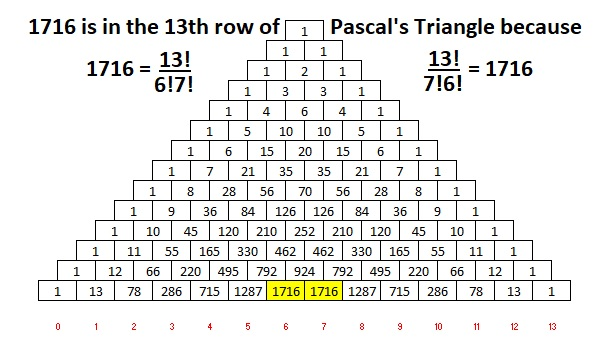# 1715 A Lot More of a Subtraction Distraction

### Today’s Puzzle:

Last time I published a puzzle with the last clue missing. Leaving out the first or the last clue only makes the puzzle slightly more difficult. What if I left out a clue more in the middle of the puzzle. I gave that some thought and designed today’s puzzle. I soon realized that I had to let you know that the 12 is one of the last eight boxes. There is only one solution. Can you find it?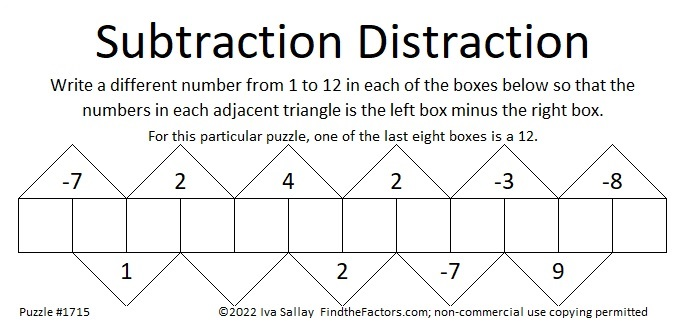I posted a solution video for it on Twitter:

### Factors of 1715:

• 1715 is a composite number.
• Prime factorization: 1715 = 5 × 7 × 7 × 7, which can be written 1715 = 5 × 7³.
• 1715 has at least one exponent greater than 1 in its prime factorization so √1715 can be simplified. Taking the factor pair from the factor pair table below with the largest square number factor, we get √1715 = (√49)(√35) = 7√35.
• The exponents in the prime factorization are 1 and 3. Adding one to each exponent and multiplying we get (1 + 1)(3 + 1) = 2 × 4 = 8. Therefore 1715 has exactly 8 factors.
• The factors of 1715 are outlined with their factor pairs in the graphic below.### More About the number 1715:

1715 = 1·7³·1·5. Thank you, OEIS.org for that fun fact!

1715 is the hypotenuse of a Pythagorean triple:
1029-1372-1715, which is (3-4-5) times 343.

1715 = 5·7³.
5·7º = 2² + 1².
5·7¹ cannot be written as the sum of two squares.
5·7² = 14² + 7².
5·7³ cannot be written as the sum of two squares.
5·7⁴ = 98² + 49².

What do you notice? What do you wonder?

# 1714 Will the Factors in This Puzzle Give You Fits?

### Today’s Puzzle:

12 and 24 have several common factors, but only one of them works in this puzzle. Will it be 2, 3, 4, 6, or 12?

What about 40 and 60’s common factors?

Don’t guess which factor to use. Start elsewhere in the puzzle where there’s only one possible common factor. Then use logic to eliminate some of the factor possibilities for 12, 24 and 40, 60. You will have to think, but it won’t be too difficult.### Factors of 1714:

• 1714 is a composite number.
• Prime factorization: 1714 = 2 × 857.
• 1714 has no exponents greater than 1 in its prime factorization, so √1714 cannot be simplified.
• The exponents in the prime factorization are 1 and 1. Adding one to each exponent and multiplying we get (1 + 1)(1 + 1) = 2 × 2 = 4. Therefore 1714 has exactly 4 factors.
• The factors of 1714 are outlined with their factor pair partners in the graphic below.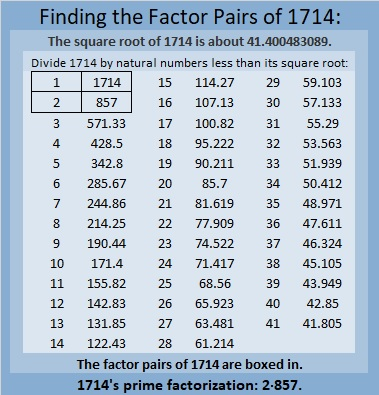### More About the Number 1714:

1714 is the sum of two squares:
33² + 25² = 1714.

1714 is the hypotenuse of a Pythagorean triple:
464-1650-1714, calculated from 33² – 25², 2(33)(25), 33² + 25².
It is also 2 times (232-825-857).

# 1713 A Little More of a Subtraction Distraction

### Today’s Puzzle:

It occurred to me that as long as the last box is neither 1 nor 12 that I could leave the clue above it blank. Can you still solve the puzzle?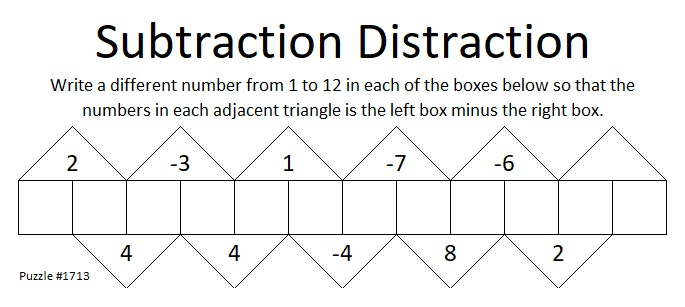### Factors of 1713:

• 1713 is a composite number.
• Prime factorization: 1713 = 3 × 571.
• 1713 has no exponents greater than 1 in its prime factorization, so √1713 cannot be simplified.
• The exponents in the prime factorization are 1 and 1. Adding one to each exponent and multiplying we get (1 + 1)(1 + 1) = 2 × 2 = 4. Therefore 1713 has exactly 4 factors.
• The factors of 1713 are outlined with their factor pair partners in the graphic below.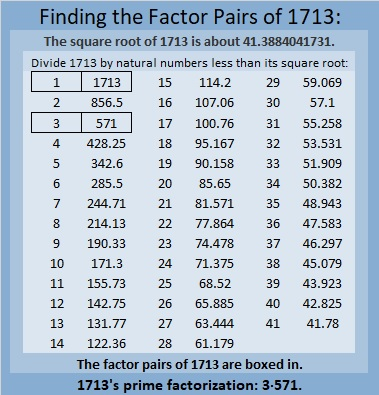### More About the Number 1713:

1713 is the difference of two squares in two different ways:
857² – 856² = 1713, and
287² – 284² = 1713.

1713 is the sum of two, three, and six consecutive numbers:
856 + 857 = 1713,
570 + 571 + 572 = 1713, and
283 + 284 + 285 + 286 + 287 + 288 = 1713.

Do you see any relationship between those two facts?

# 1712 Can You Make the Factors Fit?

### Today’s Puzzle:

This Factor Fits puzzle starts off fairly easy before it potentially might give you fits trying to place the rest of the factors. Are you game?### Factors of 1712:

• 1712 is a composite number.
• Prime factorization: 1712 = 2 × 2 × 2 × 2 × 107, which can be written 1712 = 2⁴ × 107.
• 1712 has at least one exponent greater than 1 in its prime factorization so √1712 can be simplified. Taking the factor pair from the factor pair table below with the largest square number factor, we get √1712 = (√16)(√107) = 4√107.
• The exponents in the prime factorization are 4 and 1. Adding one to each exponent and multiplying we get (4 + 1)(1 + 1) = 5 × 2 = 10. Therefore 1712 has exactly 10 factors.
• The factors of 1712 are outlined with their factor pair partners in the graphic below.### More About the Number 1712:

1712 is the difference of two squares in three different ways:
429² – 427² = 1712,
216² – 212² = 1712, and
111² – 103² = 1712.